# Friday Drag and drop proofs Draw the diagram

• Slides: 8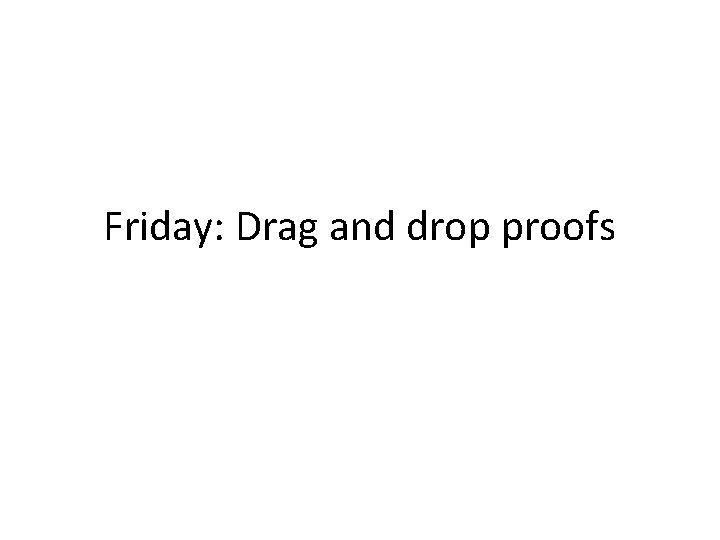Friday: Drag and drop proofs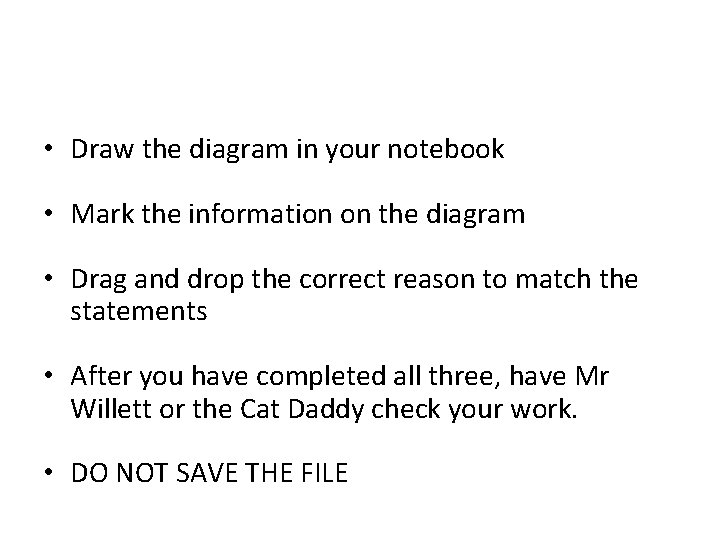• Draw the diagram in your notebook • Mark the information on the diagram • Drag and drop the correct reason to match the statements • After you have completed all three, have Mr Willett or the Cat Daddy check your work. • DO NOT SAVE THE FILE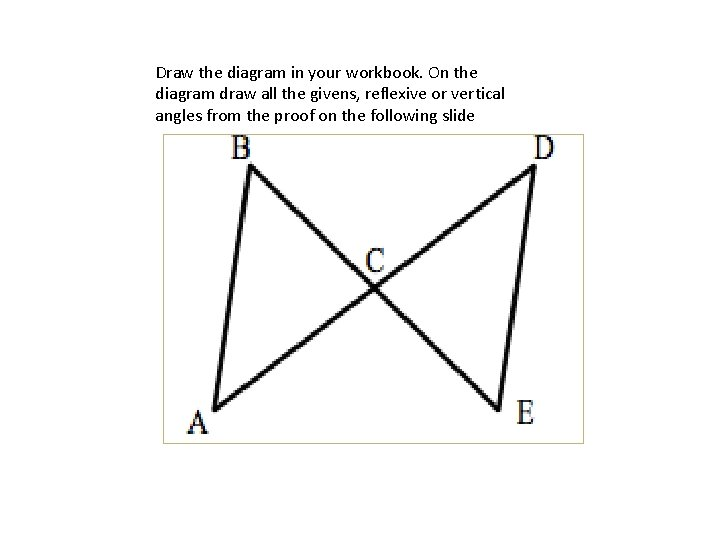Draw the diagram in your workbook. On the diagram draw all the givens, reflexive or vertical angles from the proof on the following slide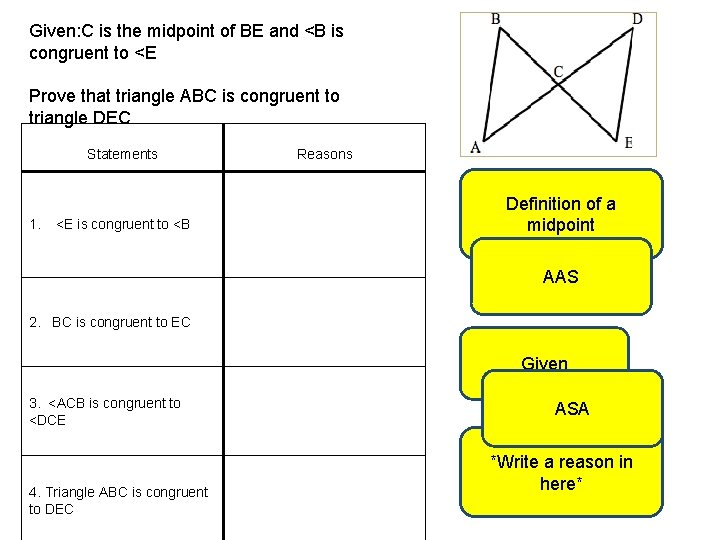Given: C is the midpoint of BE and <B is congruent to <E Prove that triangle ABC is congruent to triangle DEC Statements 1. <E is congruent to <B Reasons Definition of a midpoint AAS 2. BC is congruent to EC Given 3. <ACB is congruent to <DCE 4. Triangle ABC is congruent to DEC ASA *Write a reason in here*Draw the diagram in your workbook. On the diagram draw all the givens, reflexive or vertical angles from the proof on the following slide R S T V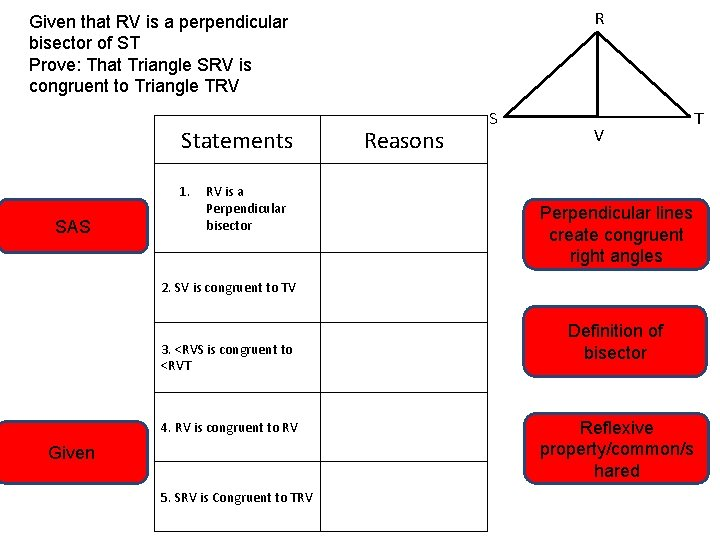R Given that RV is a perpendicular bisector of ST Prove: That Triangle SRV is congruent to Triangle TRV Statements 1. SAS RV is a Perpendicular bisector Reasons S V Perpendicular lines create congruent right angles 2. SV is congruent to TV 3. <RVS is congruent to <RVT 4. RV is congruent to RV Given 5. SRV is Congruent to TRV Definition of bisector Reflexive property/common/s hared TDraw the diagram in your workbook. On the diagram draw all the givens, reflexive or vertical angles from the proof on the following slideGiven: DB is a bisector of angle ABC and AB is congruent to BC Prove: Triangle ABD is congruent to triangle CBD Statements 1. AB is congruent to BC 2. <ABD is congruent to <CBD Reasons Definition of an angle Bisector Reflexive property Given 3. BD is congruent to BD HL 4. Triangle ABD is congruent to Triangle CBD SAS